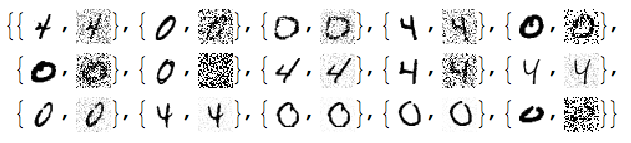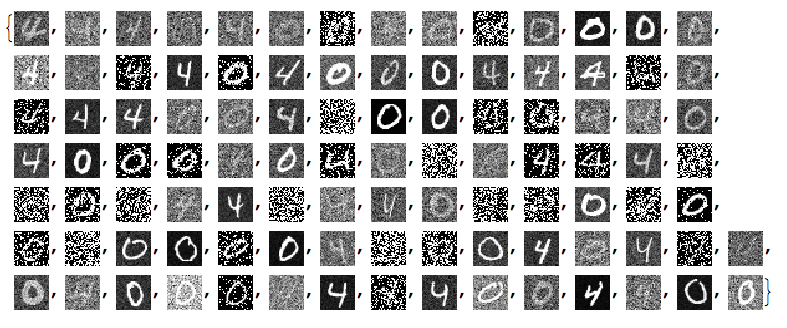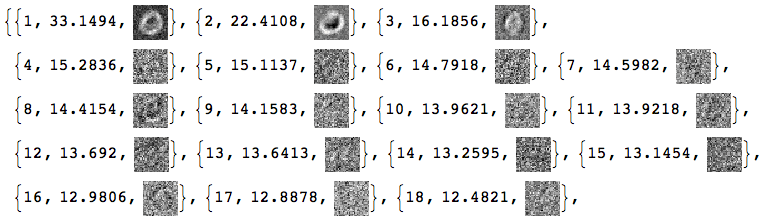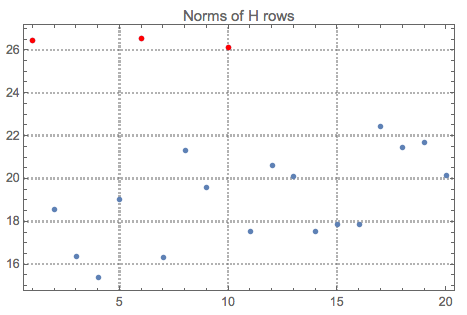# Comparison of PCA and NNMF over image de-noising

This post compares two dimension reduction techniques Principal Component Analysis (PCA) / Singular Value Decomposition (SVD) and Non-Negative Matrix Factorization (NNMF) over a set of images with two different classes of signals (two digits below) produced by different generators and overlaid with different types of noise.

PCA/SVD produces somewhat good results, but NNMF often provides great results. The factors of NNMF allow interpretation of the basis vectors of the dimension reduction, PCA in general does not. This can be seen in the example below.

## Data

First let us get some images. I am going to use the MNIST dataset for clarity. (And because I experimented with similar data some time ago — see Classification of handwritten digits.).

``````MNISTdigits = ExampleData[{"MachineLearning", "MNIST"}, "TestData"];
{testImages, testImageLabels} =
Transpose[List @@@ RandomSample[Cases[MNISTdigits, HoldPattern[(im_ -> 0 | 4)]], 100]];
testImages
``````See the breakdown of signal classes:

``````Tally[testImageLabels]
(* {{4, 48}, {0, 52}} *)``````

Verify the images have the same sizes:

``````Tally[ImageDimensions /@ testImages]
dims = %[[1, 1]]``````

Add different kinds of noise to the images:

``````noisyTestImages6 =
Table[ImageEffect[
testImages[[i]],
{RandomChoice[{"GaussianNoise", "PoissonNoise", "SaltPepperNoise"}], RandomReal}], {i, Length[testImages]}];
``````Since the important values of the signals are 0 or close to 0 we negate the noisy images:

``````negNoisyTestImages6 = ImageAdjust@*ColorNegate /@ noisyTestImages6
``````## Linear vector space representation

We unfold the images into vectors and stack them into a matrix:

``````noisyTestImagesMat = (Flatten@*ImageData) /@ negNoisyTestImages6;
Dimensions[noisyTestImagesMat]

(* {100, 784} *)
``````

Here is centralized version of the matrix to be used with PCA/SVD:

``````cNoisyTestImagesMat =
Map[# - Mean[noisyTestImagesMat] &, noisyTestImagesMat];
``````

(With NNMF we want to use the non-centralized one.)

Here confirm the values in those matrices:

``````Grid[{Histogram[Flatten[#], 40, PlotRange -> All,
ImageSize -> Medium] & /@ {noisyTestImagesMat,
cNoisyTestImagesMat}}]
``````## SVD dimension reduction

For more details see the previous answers.

``````{U, S, V} = SingularValueDecomposition[cNoisyTestImagesMat, 100];
ListPlot[Diagonal[S], PlotRange -> All, PlotTheme -> "Detailed"]
dS = S;
Do[dS[[i, i]] = 0, {i, Range[10, Length[S], 1]}]
newMat = U.dS.Transpose[V];
denoisedImages =
Map[Image[Partition[# + Mean[noisyTestImagesMat], dims[]]] &, newMat];
``````Here are how the new basis vectors look like:

``````Take[#, 50] &@
Dimensions[V][]], Diagonal[S], Transpose[V]}]
``````Basically, we cannot tell much from these SVD basis vectors images.

Here we load the packages for what is computed next:

``````Import["https://raw.githubusercontent.com/antononcube/MathematicaForPrediction/master/NonNegativeMatrixFactorization.m"]

Import["https://raw.githubusercontent.com/antononcube/MathematicaForPrediction/master/OutlierIdentifiers.m"]
``````

The blog post “Statistical thesaurus from NPR podcasts” discusses an example application of NNMF and has links to documents explaining the theory behind the NNMF utilization.

The outlier detection package is described in a previous blog post “Outlier detection in a list of numbers”.

## NNMF

This command factorizes the image matrix into the product W H :

``````{W, H} = GDCLS[noisyTestImagesMat, 20, "MaxSteps" -> 200];
{W, H} = LeftNormalizeMatrixProduct[W, H];

Dimensions[W]
Dimensions[H]

(* {100, 20} *)
(* {20, 784} *)
``````

The rows of H are interpreted as new basis vectors and the rows of W are the coordinates of the images in that new basis. Some appropriate normalization was also done for that interpretation. Note that we are using the non-normalized image matrix.

Let us see the norms of \$H\$ and mark the top outliers:

``````norms = Norm /@ H;
ListPlot[norms, PlotRange -> All, PlotLabel -> "Norms of H rows",
PlotTheme -> "Detailed"] //
ColorPlotOutliers[TopOutliers@*HampelIdentifierParameters]
OutlierPosition[norms, TopOutliers@*HampelIdentifierParameters]
OutlierPosition[norms, TopOutliers@*SPLUSQuartileIdentifierParameters]
``````Here is the interpretation of the new basis vectors (the outliers are marked in red):

``````MapIndexed[{#2[], Norm[#], Image[Partition[#, dims[]]]} &, H] /. (# -> Style[#, Red] & /@
OutlierPosition[norms, TopOutliers@*HampelIdentifierParameters])
``````Using only the outliers of \$H\$ let us reconstruct the image matrix and the de-noised images:

``````pos = {1, 6, 10}
dHN = Total[norms]/Total[norms[[pos]]]*
DiagonalMatrix[
ReplacePart[ConstantArray[0, Length[norms]],
Map[List, pos] -> 1]];
newMatNNMF = W.dHN.H;
denoisedImagesNNMF =
Map[Image[Partition[#, dims[]]] &, newMatNNMF];
``````

Often we cannot just rely on outlier detection and have to hand pick the basis for reconstruction. (Especially when we have more than one classes of signals.)

## Comparison

At this point we can plot all images together for comparison:

``````imgRows =
Transpose[{testImages, noisyTestImages6,
With[{ncol = 5},
Grid[Prepend[Partition[imgRows, ncol],
Style[#, Blue, FontFamily -> "Times"] & /@
Table[{"original", "noised", "SVD", "NNMF"}, ncol]]]]
``````We can see that NNMF produces cleaner images. This can be also observed/confirmed using threshold binarization — the NNMF images are much cleaner.

``````imgRows =
With[{th = 0.5},
Binarize[#4, th]} &, {testImageLabels, noisyTestImages6,
With[{ncol = 5},
Grid[Prepend[Partition[imgRows, ncol],
Style[#, Blue, FontFamily -> "Times"] & /@
Table[{"label", "noised", "SVD", "NNMF"}, ncol]]]]
``````Usually with NNMF in order to get good results we have to do more that one iteration of the whole factorization and reconstruction process. And of course NNMF is much slower. Nevertheless, we can see clear advantages of NNMF’s interpretability and leveraging it.

## Gallery with other experiments

In those experiments I had to hand pick the NNMF basis used for the reconstruction. Using outlier detection without supervision would not produce good results most of the time.

## Further comparison with Classify

We can further compare the de-noising results by building signal (digit) classifiers and running them over the de-noised images.

For such a classifier we have to decide:

1. do we train only with images of the two signal classes or over a larger set of signals;
2. how many signals we train with;
3. with what method the classifiers are built.

Below I use the default method of `Classify` with all digit images in MNIST that are not in the noised images set. The classifier is run with the de-noising traced these 0-4 images:

Get the images:

``````{trainImages, trainImageLabels} = Transpose[List @@@ Cases[MNISTdigits, HoldPattern[(im_ -> _)]]];
pred = Map[! MemberQ[testImages, #] &, trainImages];
trainImages = Pick[trainImages, pred];
trainImageLabels = Pick[trainImageLabels, pred];
Tally[trainImageLabels]

(* {{0, 934}, {1, 1135}, {2, 1032}, {3, 1010}, {4, 928}, {5, 892}, {6, 958}, {7, 1028}, {8, 974}, {9, 1009}} *)``````
``` ```

Build the classifier:

``````digitCF = Classify[trainImages -> trainImageLabels]
``````
``` ```

Compute classifier measurements for the original, the PCA de-noised, and NNMF de-noised images:

``````origCM = ClassifierMeasurements[digitCF, Thread[(testImages) -> testImageLabels]]

pcaCM = ClassifierMeasurements[digitCF,

nnmfCM = ClassifierMeasurements[digitCF,
``` ```

Tabulate the classification measurements results:

``````Grid[{{"Original", "PCA", "NNMF"},
{Row[{"Accuracy:", origCM["Accuracy"]}], Row[{"Accuracy:", pcaCM["Accuracy"]}], Row[{"Accuracy:", nnmfCM["Accuracy"]}]},
{origCM["ConfusionMatrixPlot"], pcaCM["ConfusionMatrixPlot"], nnmfCM["ConfusionMatrixPlot"]}},
Dividers -> {None, {True, True, False}}]``````
``` ```## 5 thoughts on “Comparison of PCA and NNMF over image de-noising”

1.Antonin Sulc says:

Hi Anton,

as long as I know there are two additional methods for image denoinsing which might be interesting for comparison. The first are dictionaries and sparse codes, where you estimate finite dictionary D based on image patches with specific size and then by enforcing sparsity (\$L_1\$, otherwise you loose convexity) on each image patch x_i by minimizing following functional
E(u) = ||x – D * a || + ||a||_1

you alter between optimizing the dictionary D and sparse codes \$a\$ (which are specific for each patch) which are linear combination of patches from dictionary D

In your case I see digits from MNIST, so you can let say create dictionary of 50 elements to have roughly 5 cases of the same letter.

More interesting is Total Variation (TV) denoising (there more variants, but \$L_1\$ is particularly nice due to convexity) where you minimize the functional for u with observed image f
||u – f||_2 + ||\nabla u||_p
The first term enforces similarity to original image, and the second term enforces the sparsity (p = 0 would be of course very nice, but it is not convex anymore, p=1 is convex and moreless enforces sparisty and p=2 over-smooths results.

If you look closer on the second term then for p=1 it prefers piecewise solutions. Very interesting results for denosing are also Total Generalized Variation, but it is beyond time I have now.

Btw. very nice topic 😉

2.Anton Antonov Antonov says: